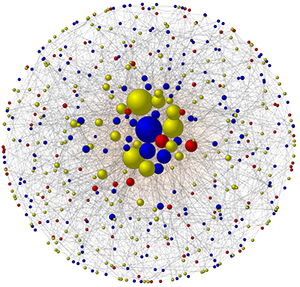This website uses features that are not well-supported by your browser. Please consider upgrading to a browser and version that fully supports CSS Grid and the CSS Flexible Box Layout Module.
Applied and Computational MathematicsResearch by Xingru Chen and Feng Fu on the effects of social networks on vaccination behavior The research of our ACM group is focused on studying real-world problems using a wide range of mathematical and computational tools, ranging from numerical methods to network science to evolutionary dynamics.

### Members

Feng Fu
Mathematical biology; Networks; Evolutionary dynamics; Cooperation; Cancer; Infectious diseases
Anne Gelb
Numerical analysis; Imaging and signal processing applications; Numerical partial differential equations
Dimitris Giannakis
Dynamical systems; Data science; Quantum information; Climate dynamics
Yoonsang Lee
Applied and Computational Mathematics; Numerical PDEs; Uncertainty quantification; Data assimilation
Ethan Levien
Probability theory and stochastic processes; Mathematical biology; Statistics
Peter Mucha
Complex systems; Data analysis; Networks
Scott Pauls
Differential geometry; Data analysis; Complex systems; Applications to economics, neuroscience, sociology, and political science
Dan Rockmore
Complex systems; Machine learning; Networks; Computational harmonic analysis
Dorothy Wallace
Mathematical biology; Ordinary differential equations; Number theory
John Voight
Number theory; Arithmetic geometry; Algebraic computing